Figure Matrix Quiz II, Non Verbal Reasoning

# Figure Matrix Quiz II, Non Verbal Reasoning - SSC CGL

Test Description

## 15 Questions MCQ Test Non Verbal Reasoning - Figure Matrix Quiz II, Non Verbal Reasoning

Figure Matrix Quiz II, Non Verbal Reasoning for SSC CGL 2023 is part of Non Verbal Reasoning preparation. The Figure Matrix Quiz II, Non Verbal Reasoning questions and answers have been prepared according to the SSC CGL exam syllabus.The Figure Matrix Quiz II, Non Verbal Reasoning MCQs are made for SSC CGL 2023 Exam. Find important definitions, questions, notes, meanings, examples, exercises, MCQs and online tests for Figure Matrix Quiz II, Non Verbal Reasoning below.
Solutions of Figure Matrix Quiz II, Non Verbal Reasoning questions in English are available as part of our Non Verbal Reasoning for SSC CGL & Figure Matrix Quiz II, Non Verbal Reasoning solutions in Hindi for Non Verbal Reasoning course. Download more important topics, notes, lectures and mock test series for SSC CGL Exam by signing up for free. Attempt Figure Matrix Quiz II, Non Verbal Reasoning | 15 questions in 30 minutes | Mock test for SSC CGL preparation | Free important questions MCQ to study Non Verbal Reasoning for SSC CGL Exam | Download free PDF with solutions
 1 Crore+ students have signed up on EduRev. Have you?
Figure Matrix Quiz II, Non Verbal Reasoning - Question 1

### Directions to Solve In each of the following questions, find out which of the answer figures (1), (2), (3) and (4) completes the figure matrix ? Question - Select a suitable figure from the four alternatives that would complete the figure matrix.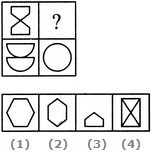Detailed Solution for Figure Matrix Quiz II, Non Verbal Reasoning - Question 1
The two parts of the first figure are rearranged and joined along the longer sides. The common side is then lost to form the second figure.
Figure Matrix Quiz II, Non Verbal Reasoning - Question 2

### Directions to Solve In each of the following questions, find out which of the answer figures (1), (2), (3) and (4) completes the figure matrix ? Question - Select a suitable figure from the four alternatives that would complete the figure matrix.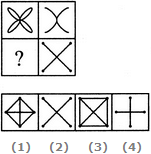Detailed Solution for Figure Matrix Quiz II, Non Verbal Reasoning - Question 2
The second figure is a part of the first figure (but is not exactly the same as the first figure).
Figure Matrix Quiz II, Non Verbal Reasoning - Question 3

### Directions to Solve In each of the following questions, find out which of the answer figures (1), (2), (3) and (4) completes the figure matrix ? Question - Select a suitable figure from the four alternatives that would complete the figure matrix.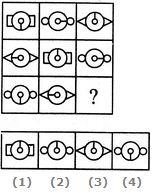Detailed Solution for Figure Matrix Quiz II, Non Verbal Reasoning - Question 3
In each row, the central part of the first figure rotates either 90o CW or 90o ACW to form the central part of the second figure and the central part of the first figure rotates through 180o to form the central part of the third figure. Also, in each row, there are 3 types of side elements - rectangles, circles and triangles.
Figure Matrix Quiz II, Non Verbal Reasoning - Question 4

In each of the following questions, find out which of the answer figures (1), (2), (3) and (4) completes the figure matrix ?

Question -

Select a suitable figure from the four alternatives that would complete the figure matrix.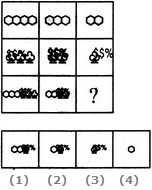Detailed Solution for Figure Matrix Quiz II, Non Verbal Reasoning - Question 4
In each column, the third figure (lowermost figure) contains one less number of hexagons as the first figure (uppermost figure) and the same number of trees as the second figure (middle figure).
Figure Matrix Quiz II, Non Verbal Reasoning - Question 5

In each of the following questions, find out which of the answer figures (1), (2), (3) and (4) completes the figure matrix ?

Question -

Select a suitable figure from the four alternatives that would complete the figure matrix.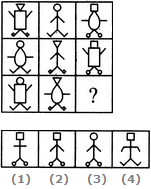Detailed Solution for Figure Matrix Quiz II, Non Verbal Reasoning - Question 5
There are 3 types of faces, 3 types of bodies, 3 types of hands and 3 types of legs, each of which is used only once in a single row. So, the features which have not been used in the first two figures of the third row would combine to produce the missing figure.
Figure Matrix Quiz II, Non Verbal Reasoning - Question 6

In each of the following questions, find out which of the answer figures (1), (2), (3) and (4) completes the figure matrix ?

Question -

Select a suitable figure from the four alternatives that would complete the figure matrix.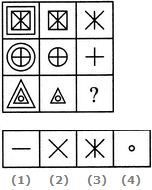Detailed Solution for Figure Matrix Quiz II, Non Verbal Reasoning - Question 6
In each row, the second figure is obtained by removing the outermost element of the first figure and the third figure is obtained by removing the outermost element of the second figure.
Figure Matrix Quiz II, Non Verbal Reasoning - Question 7

In each of the following questions, find out which of the answer figures (1), (2), (3) and (4) completes the figure matrix ?

Question -

Select a suitable figure from the four alternatives that would complete the figure matrix.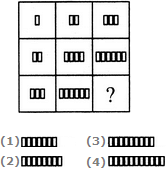Detailed Solution for Figure Matrix Quiz II, Non Verbal Reasoning - Question 7
In each row (as well as in each column), the number of rectangles in the second figure is two times the number of rectangles in the first figure and the number of rectangles in the third figure is three times the number of rectangles in the first figure.
Figure Matrix Quiz II, Non Verbal Reasoning - Question 8

In each of the following questions, find out which of the answer figures (1), (2), (3) and (4) completes the figure matrix ?

Question -

Select a suitable figure from the four alternatives that would complete the figure matrix.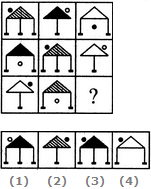Detailed Solution for Figure Matrix Quiz II, Non Verbal Reasoning - Question 8
There are 3 types of shadings in the triangles, 3 types of legs, 3 positions of circles, each of which is used only once in a single row. The circle is shaded in alternate figures.
Figure Matrix Quiz II, Non Verbal Reasoning - Question 9

In each of the following questions, find out which of the answer figures (1), (2), (3) and (4) completes the figure matrix ?

Question -

Select a suitable figure from the four alternatives that would complete the figure matrix.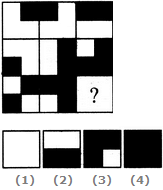Detailed Solution for Figure Matrix Quiz II, Non Verbal Reasoning - Question 9
In each row (as well as each column), the third figure is a combination of all the, elements of the first and the second figures
Figure Matrix Quiz II, Non Verbal Reasoning - Question 10

In each of the following questions, find out which of the answer figures (1), (2), (3) and (4) completes the figure matrix ?

Question -

Select a suitable figure from the four alternatives that would complete the figure matrix.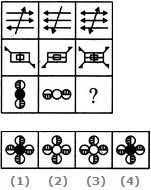Detailed Solution for Figure Matrix Quiz II, Non Verbal Reasoning - Question 10
In each row, the third figure is a combination of the first and the second figure.
Figure Matrix Quiz II, Non Verbal Reasoning - Question 11

In each of the following questions, find out which of the answer figures (1), (2), (3) and (4) completes the figure matrix ?

Question -

Select a suitable figure from the four alternatives that would complete the figure matrix.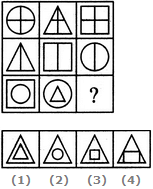Detailed Solution for Figure Matrix Quiz II, Non Verbal Reasoning - Question 11
In each row, the triangle follows the circle, the square follows the triangle and the circle follows the square. In case of the third row, the above rule exists for the inner as well as the outer elements.
Figure Matrix Quiz II, Non Verbal Reasoning - Question 12

In each of the following questions, find out which of the answer figures (1), (2), (3) and (4) completes the figure matrix ?

Question -

Select a suitable figure from the four alternatives that would complete the figure matrix.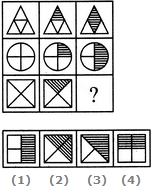Detailed Solution for Figure Matrix Quiz II, Non Verbal Reasoning - Question 12
In each row, the second figure is obtained by shading one of the four parts of the first figure and the third figure is obtained by shading two out of the four parts of the first figure.
Figure Matrix Quiz II, Non Verbal Reasoning - Question 13

In each of the following questions, find out which of the answer figures (1), (2), (3) and (4) completes the figure matrix ?

Question -

Select a suitable figure from the four alternatives that would complete the figure matrix.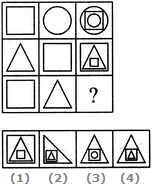Detailed Solution for Figure Matrix Quiz II, Non Verbal Reasoning - Question 13
In each row, the second figure forms the innermost and the outermost elements of the third figure and the first figure forms the middle element of the third figure.
Figure Matrix Quiz II, Non Verbal Reasoning - Question 14

In each of the following questions, find out which of the answer figures (1), (2), (3) and (4) completes the figure matrix ?

Question -

Select a suitable figure from the four alternatives that would complete the figure matrix.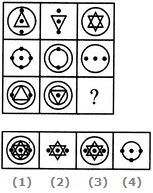Detailed Solution for Figure Matrix Quiz II, Non Verbal Reasoning - Question 14
The third figure in each row comprises of parts which are not common to the first two figures.
Figure Matrix Quiz II, Non Verbal Reasoning - Question 15

In each of the following questions, find out which of the answer figures (1), (2), (3) and (4) completes the figure matrix ?

Question -

Select a suitable figure from the four alternatives that would complete the figure matrix.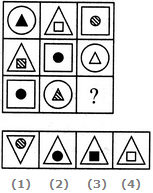Detailed Solution for Figure Matrix Quiz II, Non Verbal Reasoning - Question 15
In each row, there are three types of outer elements (circle, triangle and square), three types of inner elements (circle, triangle and square) and three types of shadings in the inner elements (black, white and lines).

## Non Verbal Reasoning

40 tests
Information about Figure Matrix Quiz II, Non Verbal Reasoning Page
In this test you can find the Exam questions for Figure Matrix Quiz II, Non Verbal Reasoning solved & explained in the simplest way possible. Besides giving Questions and answers for Figure Matrix Quiz II, Non Verbal Reasoning, EduRev gives you an ample number of Online tests for practice

40 tests